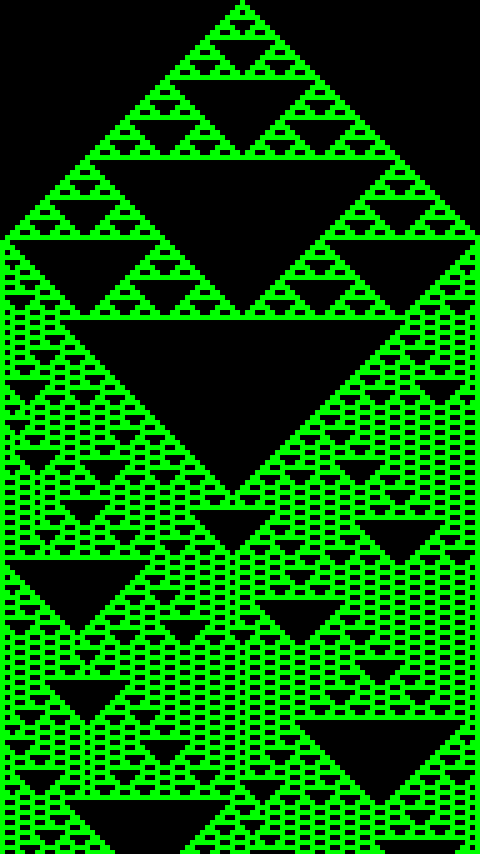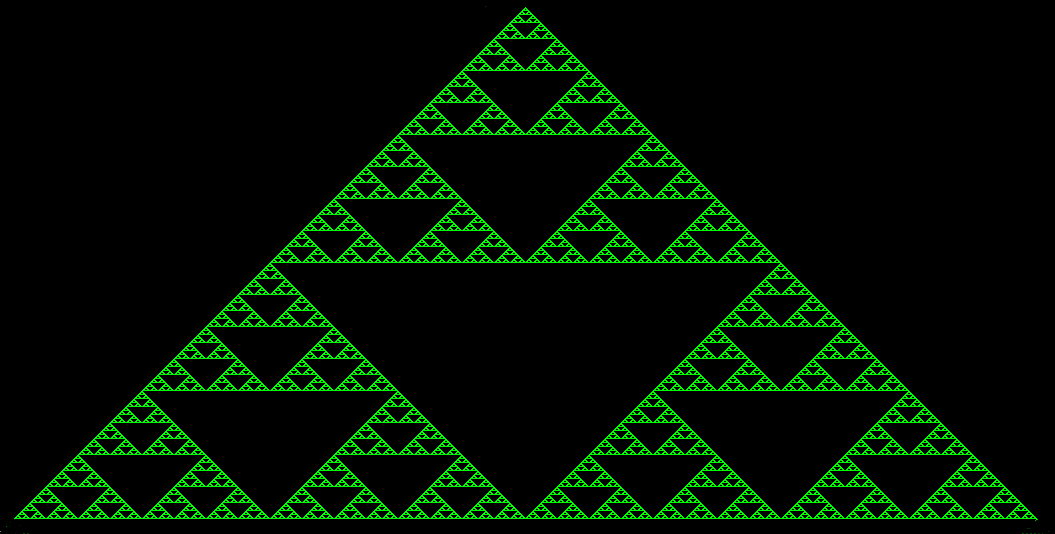### C++

• Articles
• Sierpinski Triangle Fractal - The easies
Oct 13, 2015 (last update: Oct 13, 2015)

# Sierpinski Triangle Fractal - The easiest way to produce randomness### What is Sierpinski Triangle?

Sierpinski Triangle is a group of multiple(or infinite) triangles. Just see the Sierpinski Triangle below to find out how infinite it may look.The concept behind this is the fact that the filled triangle is filled by an empty equilateral triangle in the center in such a way that this triangular space is congruent to the three triangles being formed around it.If you see my previous article which is given here, you see that there was a triangle which I just break into further triangles without a base, in a kind of brute manner. This time it will be done in a technical manner! In other words, we don't make a triangle and then, break it into three, but we will do something else in order to produce randomness as well.

The concept that we will use, is simple. It will have tiles! If there are one or more than one tiles and, not three tiles above the tile space then, we will put a tile in that space or else, no tiles!

### The Implementation

First thing, you should know basics of C++ as well as a bit of SDL2 and basic trigonometry to understand it.
We are going to use SDL2 to have some graphics. We will only use some of its basic primitive drawing methods for drawing lines. So, we are going to include only SDL2 header. This time, it will be multiple file project.

Files: main.cpp, SierpinskiTile.h, SierpinskiTile.cpp

In SierpinskiTile.h:
We need to include SDL.h for drawing and list to have a list of SDL_Rect* or tiles.

 ``12`` ``````#include #include ``````

Now, here's the class with preprocessor directives:

 ``123456789101112131415161718192021`` ``````#ifndef _SIERPINSKI_TILE_ #define _SIERPINSKI_TILE_ class SierpinskiTile { public: SierpinskiTile(int scrW, int scrH, int w, int h) : scrW(scrW), scrH(scrH), tileW(w), tileH(h) {}; ~SierpinskiTile(); void setTile(int x_index, int y_index); bool isThereTile(int x_index, int y_index); void calculate(int y_index = -1); void draw(SDL_Renderer*& renderer, int r, int g, int b, int y_index); private: int scrW, scrH; int tileW, tileH; std::list rects; }; #endif ``````

In SierpinskiTile.cpp:
Here's the implementation with some comments to have understanding:

Destructor:
 ``1234567`` ``````#include "SierpinskiTile.h" SierpinskiTile::~SierpinskiTile() //Deleting all resources in destructor { for (auto itr : rects) delete itr; }``````

setTile() method to set tile on the tile index position:
 ``12345678910`` ``````void SierpinskiTile::setTile(int x_index, int y_index) //Setting tile on the tile index position { SDL_Rect* rectToAdd = new SDL_Rect; rectToAdd->x = x_index * tileW; rectToAdd->y = y_index * tileH; rectToAdd->w = tileW; rectToAdd->h = tileH; rects.push_back(rectToAdd); }``````

isThereTile() method to find out whether given tile index position have a tile there or not:
 ``123456789`` ``````bool SierpinskiTile::isThereTile(int x_index, int y_index) //Finding out whether a tile is here or not { for (auto itr : rects) if (itr->x == tileW * x_index && itr->y == tileH * y_index) return true; return false; }``````

The most important -> calculate() method to find out tiles arrangement for the next row, default parameter is -1 which will cause calculate() method to calculate all the rows' tile arrangement:
 ``12345678910111213141516171819202122232425262728293031323334353637383940414243`` ``````void SierpinskiTile::calculate(int y_index) //Calculating where to put tiles in the next row //by the tile arrangement present in the previous row { ///////////////////////////////////////////////// //Conditions for putting a tile below the upper tile (or tile space): // 1- Tile is at that spot, 0- Tile is not at that spot, X- Unknown (can be 0 or 1) ///////////////////////////////////////////////// // Case 1: 0 1 0, Case 2: 1 0 0, Case 3: 0 0 1, // Case 4: 1 1 0, Case 5: 1 0 1, Case 6: 0 1 1 // Output for Cases 1-6: X 1 X ///////////////////////////////////////////////// // Case 7: 0 0 0, Case 8: 1 1 1 // Output for Cases 7-8: X 0 X int y = 0; if (y_index > -1) { y = y_index; for (int x = 0; x < scrW / tileW; x++) { if ((isThereTile(x, y) || isThereTile(x + 1, y) || isThereTile(x - 1, y)) && !(isThereTile(x, y) && isThereTile(x + 1, y) && isThereTile(x - 1, y)) ) setTile(x, y + 1); } } else { for (; y < scrH / tileH; y++) for (int x = 0; x < scrW / tileW; x++) { if ((isThereTile(x, y) || isThereTile(x + 1, y) || isThereTile(x - 1, y)) && !(isThereTile(x, y) && isThereTile(x + 1, y) && isThereTile(x - 1, y)) ) setTile(x, y + 1); } } }``````

The ahhh... second most important -> draw() method which actually draws only a row and deletes all the tiles in all the previous rows:
 ``123456789101112131415161718192021222324`` ``````void SierpinskiTile::draw(SDL_Renderer*& renderer, int r, int g, int b, int y_index) { SDL_SetRenderDrawColor(renderer, r, g, b, 255); //Setting renderer's color std::list deleteRects; //For getting a list of rectangles/tiles to be deleted for (auto itr : rects) { SDL_RenderFillRect(renderer, itr); //Draw all tiles present in the rects which //will be just all tiles in the particular row if (itr->y <= tileH * y_index) //Put all tiles of rows before the given row //to deleteRects for deleting deleteRects.push_back(itr); } for (auto itr : deleteRects) //Delete all collected tiles and clear them { rects.remove(itr); delete itr; } deleteRects.clear(); SDL_SetRenderDrawColor(renderer, 0, 0, 0, 255); //Resetting renderer's color }``````

In main.cpp:
First, we need some necessary constants along with SDL_Window*, SDL_Renderer* and SDL_Event. We also need a boolean as well.

 ``1234567891011121314151617`` ``````#include #include "SierpinskiTile.h" #undef main //Solution to the problem: No entry point defined. const int SCR_W = 640; const int SCR_H = 480; const int TILE_W = 5; //Each tile's width const int TILE_H = 5; //Each tile's height SDL_Window* window = NULL; SDL_Renderer* renderer = NULL; SDL_Event event; bool quit = false; SierpinskiTile* generator = NULL;``````

Time for action here, every thing is all fine and main() method is now, easy to implement!
 ``123456789101112131415161718192021222324252627282930313233343536373839404142`` ``````int main(int argc, char** args) { SDL_Init(SDL_INIT_VIDEO); //Initializing SDL2 window = SDL_CreateWindow("Koch Fractal", SDL_WINDOWPOS_UNDEFINED, SDL_WINDOWPOS_UNDEFINED, SCR_W, SCR_H, SDL_WINDOW_SHOWN); //Creating window renderer = SDL_CreateRenderer(window, -1, SDL_RENDERER_ACCELERATED); //Creating renderer SDL_SetRenderDrawColor(renderer, 0, 0, 0, 255); //Setting default screen color generator = new SierpinskiTile(SCR_W, SCR_H, TILE_W, TILE_H); //Creating fractal generator generator->setTile((SCR_W / TILE_W) / 2, 0); //Setting a tile at the top middle of the screen int row = 0; while (!quit) { while (SDL_PollEvent(&event) > 0) //Minimal event polling for proper quitting if (event.type == SDL_QUIT) quit = true; //***NOTE: Screen must not be cleaned as the draw() method draws a row only //and deletes all tiles of the previous rows*** //SDL_RenderClear(renderer); if (row < SCR_H / TILE_H) //Draw and calculate until the last row { generator->draw(renderer, 0, 255, 0, row-1); //Drawing the row in green color SDL_RenderPresent(renderer); //Updating screen generator->calculate(row++); //Calculating the next row } } delete generator; //Deallocating fractal generator SDL_DestroyRenderer(renderer); SDL_DestroyWindow(window); SDL_Quit(); //Clearing all SDL resources return 0; }``````

It's pretty much straight forward and I hope that you understand that...

### The Results

Following images show the result. It was executed on a mobile device using C4Droid, a program for running C++ programs on Android.

I hope that this excites you for more programming, algorithms and fractals. For more such things, visit my blog bacprogramming.wordpress.com.

The detailing depends on the constants TILE_W and TILE_H. The lesser the values, the more detailing the fractal shows.

Less details:-Average details:-More details:-Most details:-I ran it on PC as well. Here is another extremely detailed fractal:-### The Randomness!

With setting a tile at the top corner, we can see that the fractal produces confused and hardly understandable patterns of triangles which looks random in nature.

The same code with different setTile() at start can produce this: